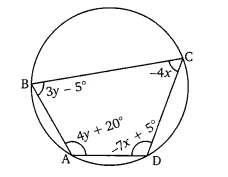# Ex.3.7 Q8 Pair of Linear Equations in Two Variables Solution - NCERT Maths Class 10

Go back to  'Ex.3.7'

## Question

$$ABCD$$ is a cyclic quadrilateral finds the angles of the cyclic quadrilateral.Video Solution
Pair Of Linear Equations In Two Variables
Ex 3.7 | Question 8

## Text Solution

What is Known?

Measurement of the angles of the cyclic quadrilateral in terms of $$x$$ and $$y.$$

What is Unknown?

Measurement of the angles of the cyclic quadrilateral.

Reasoning:

Pairs of opposite angles of a cyclic quadrilateral are supplementary.

Steps:

We know that the sum of the measures of opposite angles in a cyclic quadrilateral is $$180^\circ.$$

Therefore,

\begin{align}\angle A + \angle C &= {180^\circ}\\\left( {4y + 20} \right) + \left( { - 4x} \right) &= 180\\ 4y + 20 - 4x &= 180\\ - 4\left( {x - y} \right)& = 160\\x - y &= - 40 \qquad \left( 1 \right)\end{align}

And

\begin{align}\angle B + \angle D &= {180^\circ}\\\left( {3y - 5} \right) + \left( { - 7x + 5} \right) &= 180\\ 3y - 5 - 7x + 5 &= 180\\ - 7x + 3y &= 180\\7x - 3y &= - 180 \quad \left( 2 \right)\end{align}

Multiplying equation $$(1)$$ by $$3,$$ we obtain

$3x - 3y = - 120\qquad \left( 3 \right)$

Subtracting equation $$(3)$$ from equation $$(2),$$ we obtain

\begin{align}4x &= - 60\\x &= - 15\end{align}

Substituting $$x = - 15$$ in equation $$(1),$$ we obtain

\begin{align} - 15 - y &= - 40\\y &= 25\end{align}

Therefore,

\begin{align}\angle A &= 4 \times 25 + 20 = {120^\circ}\\\angle B &= 3 \times 25 - 5 = {70^\circ}\\ \angle C &= - 4 \times \left( { - 15} \right) = {60^\circ}\\\angle D &= - 7 \times \left( { - 15} \right) + 5 = {110^\circ}\end{align}

Learn from the best math teachers and top your exams

• Live one on one classroom and doubt clearing
• Practice worksheets in and after class for conceptual clarity
• Personalized curriculum to keep up with school